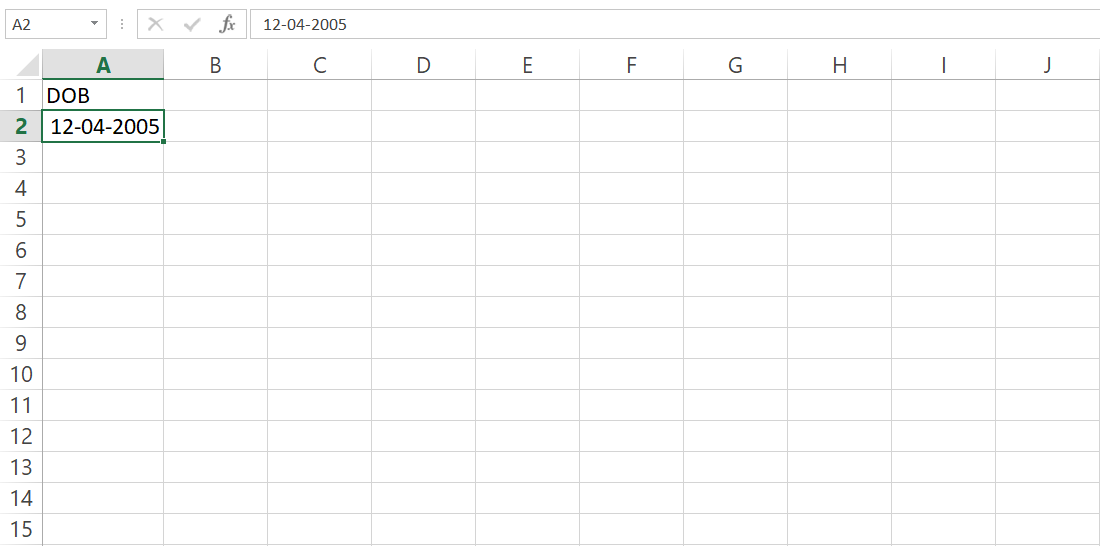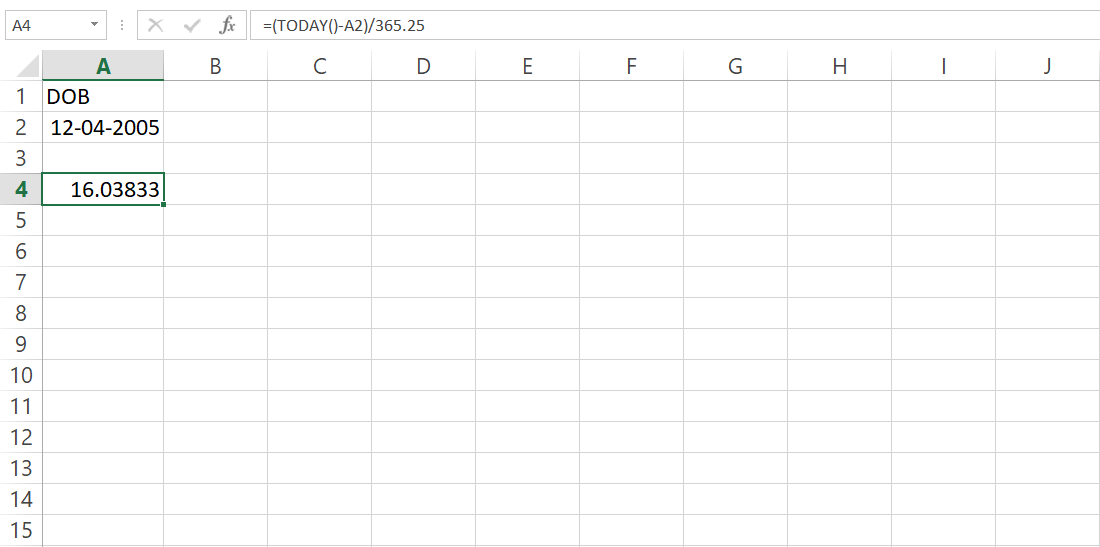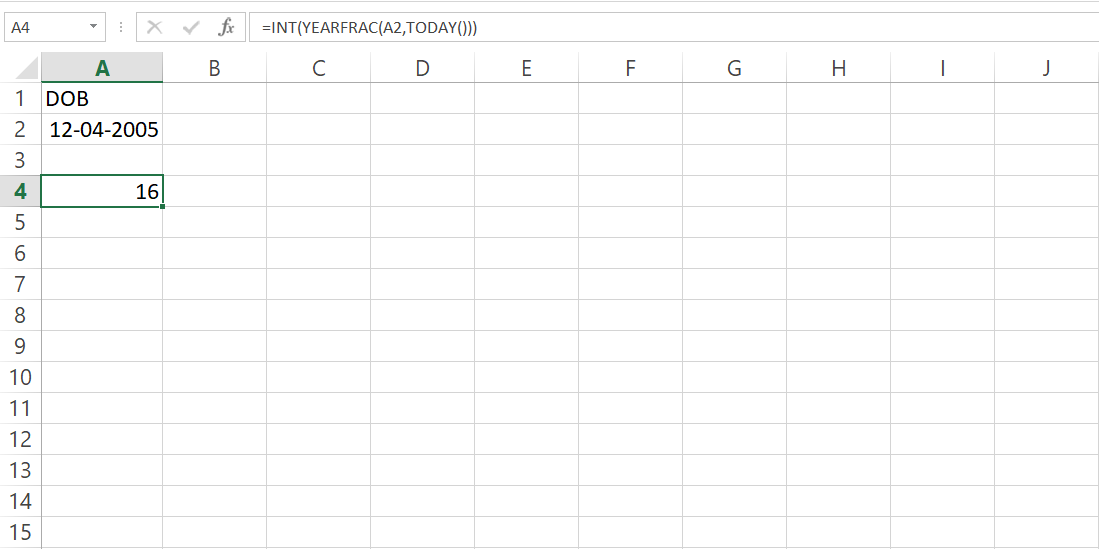GeeksforGeeks App
Open AppBrowser
Continue

# How Can We Calculate Age Without DATEDIF Function?

In this article, we’ll explore different ways of finding age in Excel, other than the usual way of finding age using DATEDIF function. For this we can use one of the two ways:

• Using simple mathematics
• Using the YEAR function

### USING SIMPLE MATH:

We can easily find age of a person using simple Math. All we need to do is, subtract the DOB from current date and divide it by 365.25

So, the formula would be:

`= (TODAY()-date_of_birth)/365.25`

Example:In this case, the formula to calculate age would be:

`=(TODAY()-A2)/365.25`With the current date of 26.04.2021, the age of above person is 16 years.

Remarks:

1. This formula returns the age in decimal form. In order to get age in integer form, we can wrap the formula in INT function. The syntax would be:

`= INT((TODAY()- date_of_birth)/365.25)`

2. This formula will produce wrong result if you try to find age of someone who hasn’t lived through a leap year.

### USING YEARFRAC FUNCTION:

YEARFRAC function in Excel returns a decimal value that represents fractional years between two dates. We can use this function to calculate age.

```Syntax:
= INT(YEARFRAC(date_of_birth, TODAY()))```

Example:My Personal Notes arrow_drop_up